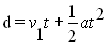### Mathematical Development of Linear Motion Equations

#### >>>The equation of motion (v2 = v1 + at) developed in the previous section is really a linear equation of the form:  y = mx + b

Where::
m is the slope of the line, and b is the y-intercept

Using our kinematics symbols we can redefine the above relationship as follows:

y = mx + b  is a liner function (a straight line) of  v(t); "velocity as a function of time" in a distance-time graph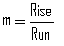recalling from previous knowledgeIn a velocity-time graph, this becomes: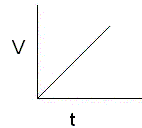More specifically:Using equations 1 and 2 we can obtain aThe above equation can also be written as d = v1t + 1/2 at2

Another useful equation can be derived as follows: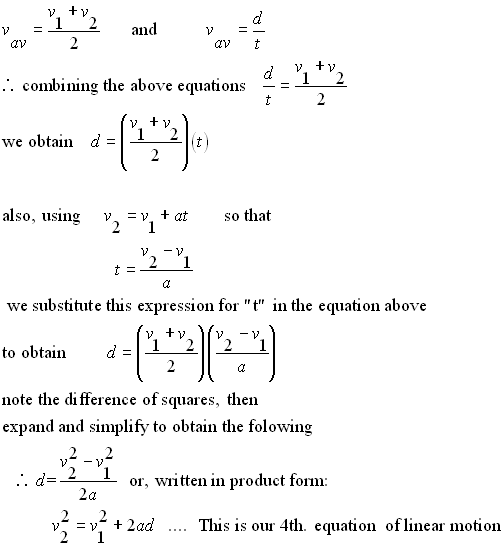1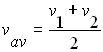2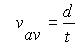3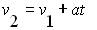4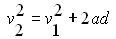5